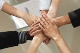# GridCalculated column question

GridTop achievementsRank 1
Kathy asked on 08 Nov 2010, 12:11 AM
I have 2 NumericColumns and one Calculated column.  The value in the Calculated column is a product of column1 and column2.  I need to apply another variable to the calculation, depending on the value in column2.  For example, if the value in column2 is 3, the product needs to be ( column1 value * column2 value * .4) and if the value in column2 is 4, the product needs to be ( column1 value * column2 value * .2).  Basically I need something conditional so that I know what the additional variable needs to be.  How would I go about doing something like this?  Thanks.

## 1 Answer, 1 is accepted

0Princy
Top achievementsRank 2
answered on 08 Nov 2010, 04:51 AM
Hello Kathy,

The following code snippet shows how to achieve this. You can access GridNumericColumn and GridCalculatedColumn value from code behind and perform necessary operation.

PX:
`<``Columns``>`
`    ``<``telerik:GridNumericColumn` `DataField``=``"Column1"` `UniqueName``=``"GridNumericColumn1"``>`
`    ``</``telerik:GridNumericColumn``>`
`    ``<``telerik:GridNumericColumn` `DataField``=``"Column2"` `UniqueName``=``"GridNumericColumn2"``>`
`    ``</``telerik:GridNumericColumn``>`
`    ``<``telerik:GridCalculatedColumn` `DataFields``=``"Column1, Column2"` `Expression``=``"{0}*{1}"`
`     ``UniqueName``=``"GridCalculatedColumn"``>`
`    ``</``telerik:GridCalculatedColumn``>`
`    ``</``telerik:GridBoundColumn``>`
`</``Columns``>`

C#:
`protected` `void` `RadGrid1_ItemDataBound(``object` `sender, GridItemEventArgs e)`
`   ``{`
`       ``if` `(e.Item ``is` `GridDataItem)`
`       ``{`
`           ``GridDataItem item = (GridDataItem)e.Item;`
`           ``Int32 column1 =Convert.ToInt32(item[``"GridNumericColumn1"``].Text);`
`           ``Int32 column2 = Convert.ToInt32(item[``"GridNumericColumn2"``].Text);`
`           ``if``(column2==3)`
`              ``item[``"GridCalculatedColumn"``].Text = (column1 * column2 *4).ToString();`
`           ``if``(column2==4)`
`               ``item[``"GridCalculatedColumn"``].Text = (column1 * column2 * 2).ToString();`
`       ``}`
`   ``}`

Thanks,
Princy.
Tags
GridRank 1Rank 2